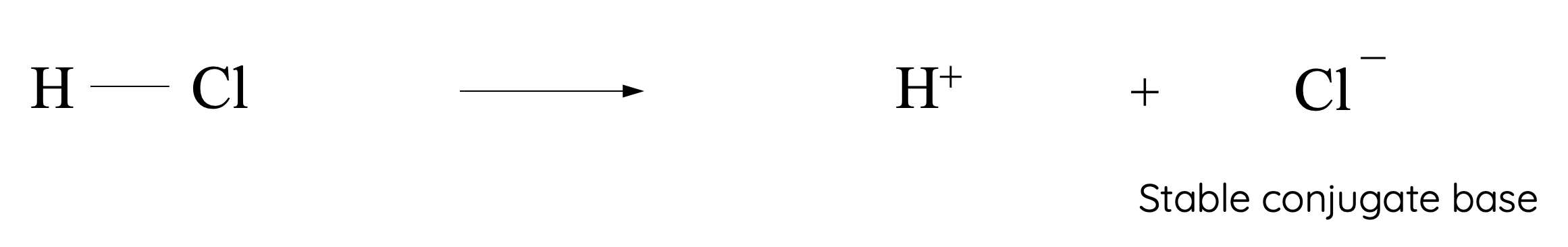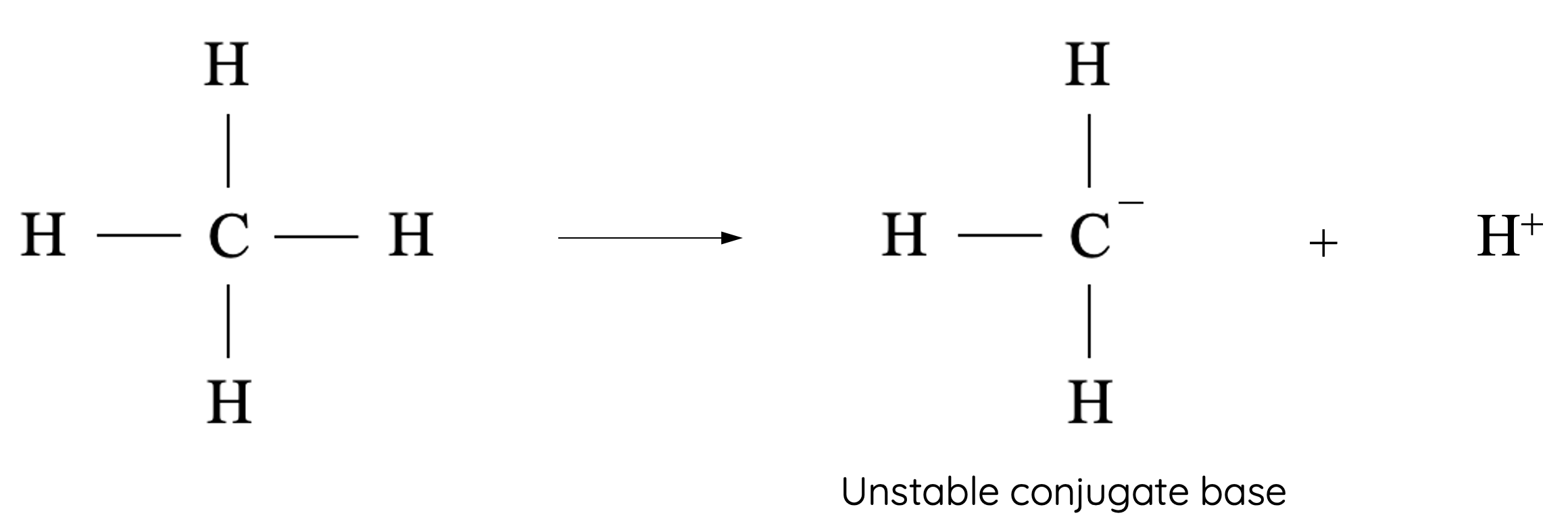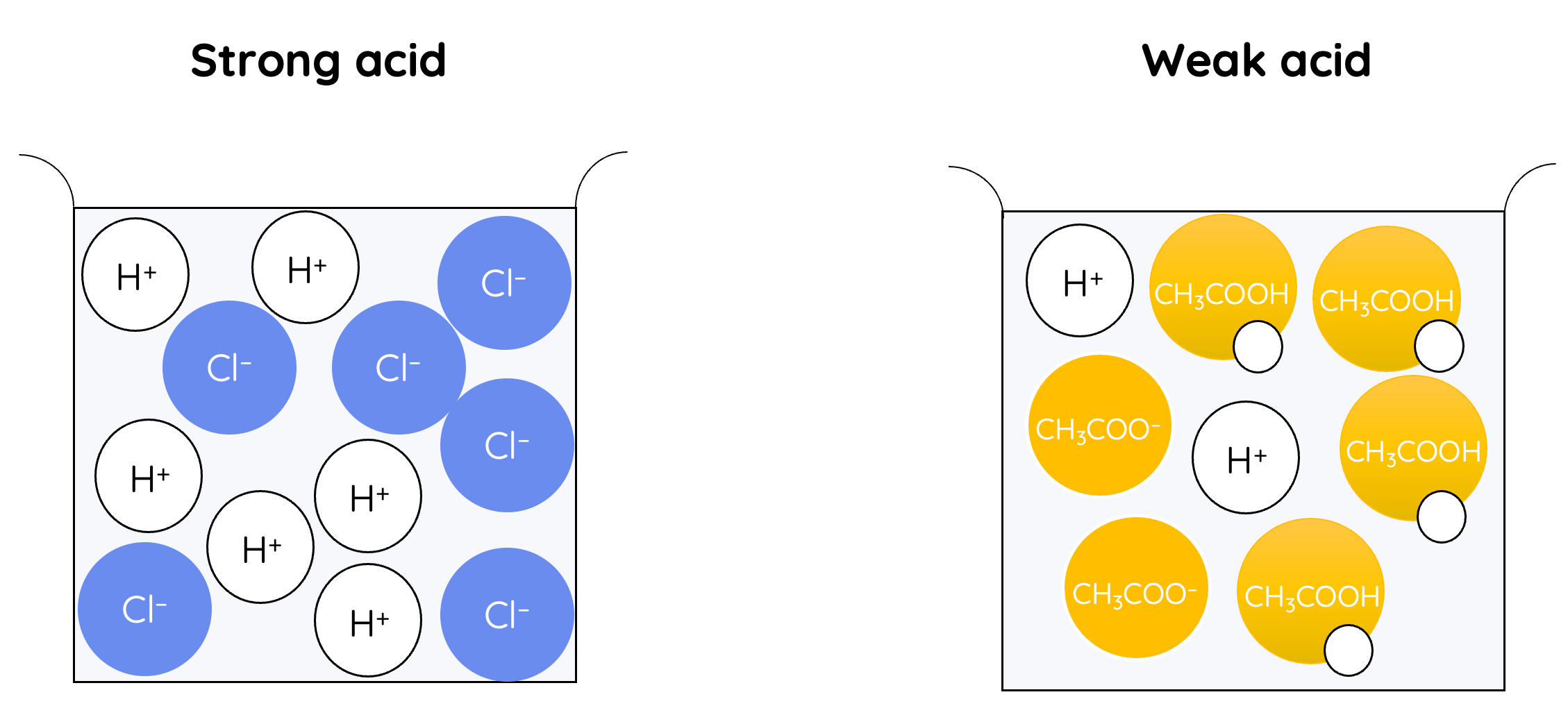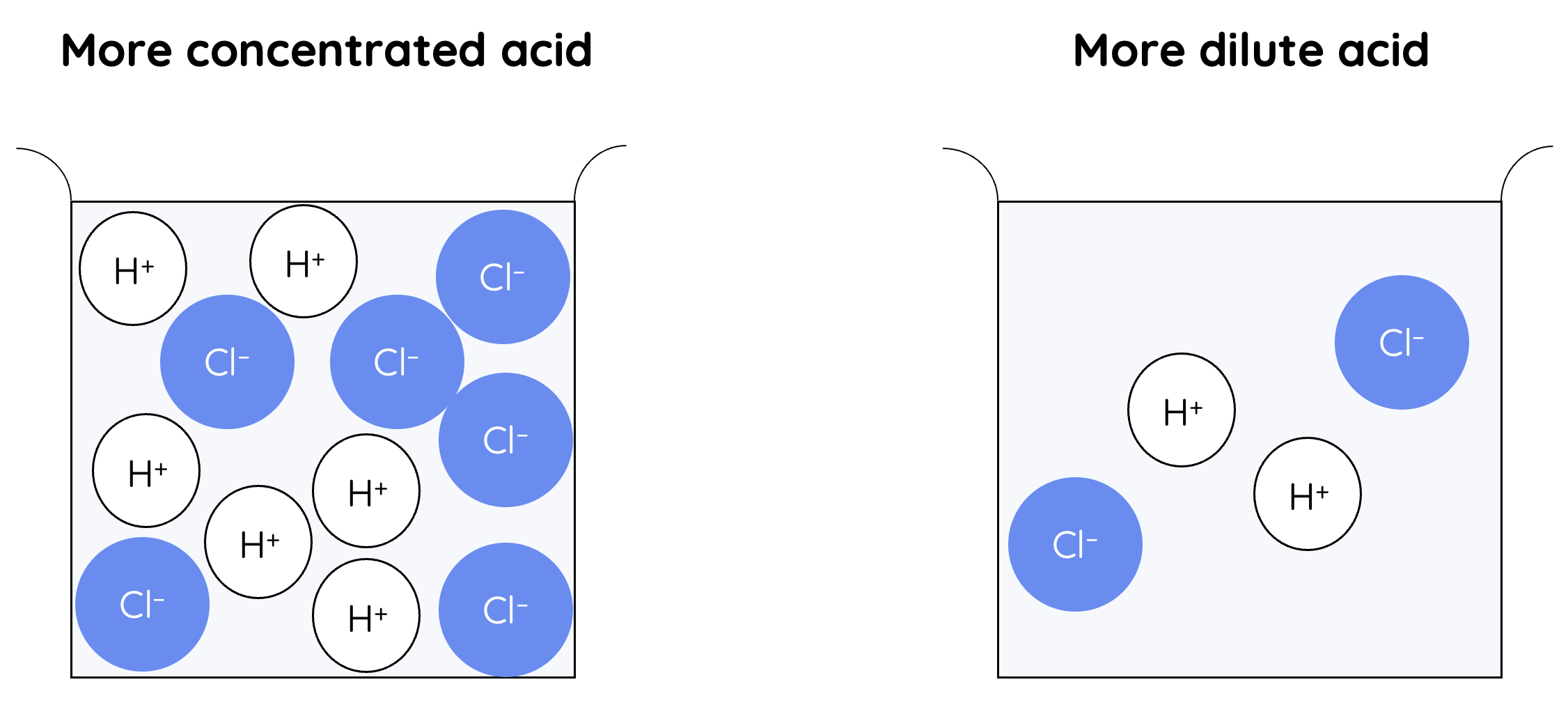# Acid and Base Strength

This is part of the HSC Chemistry course under the topic Using Brønsted-Lowry Theory.

### HSC Chemistry Syllabus

• Construct models and/or animations to communicate the differences between strong, weak, concentrated and dilute acids and bases

### What is the difference between strength and concentration of acids/bases?

This video discusses the difference between strong and weak acids/bases. The video also outlines the difference between strength and concentration.

### Brønsted-Lowry Acids and Bases

• Recall that Brønsted-Lowry theory explains that acids are substances which donate protons and bases are substances which accept protons.

$$HF(aq) + H_2O(l) \leftrightharpoons H_3O^+(aq) + F^-(aq)$$

$$NH_3(aq) + H_2O(l) \leftrightharpoons NH_4^-(aq) + OH^-(aq)$$

• In the top equation, we can see there is the formation of the hydronium ion which must have been formed from the acceptance of a proton which is donated from HF. This means that HF must be an acid

• In the bottom equation, there is the formation of an ammonium ion from the acceptance of a proton by the ammonia. This means that ammonia must be a base.

## Brønsted-Lowry Theory – Conjugate Acid and Base Pairs

The Brønsted-Lowry theory:

• defines acids as proton donors
• defines bases as proton acceptors
• is independent of the state of the reactants or the solvent
• demonstrate how acid-base reactions in reversible reactions involve the transfer of proton(s)

The theory introduces the concept of conjugate acid-base pairs:

• an acid forms a base when it loses a proton in a reversible reaction i.e. when the acid is weak
• a base forms an acid when it gains a proton in a reversible reaction
• the acid and the base it forms are called a conjugate acid-base pair
• the base and the acid it forms are called a conjugate acid-base pair

The acid and base of a conjugate acid-base pair differ from each other in structure by only 1 proton. For example, the reaction between acetic acid and water:

$$CH_3COOH(aq) + H_2O(l) \leftrightharpoons CH_3COO^-(aq) + H_3O^+(aq)$$

### Acidic Hydrogen

• A limitation to Humphrey Davy's hydrogen theory of acids was that not all hydrogen–containing molecules are acids. Molecules are only considered acids if the hydrogen atoms can be ionised or donated away.
• Acidic hydrogens are ones that can be donated or deprotonated from the molecule.• The acidity of a particular hydrogen is dependent on the stability of the conjugate base. When the conjugate base is more stable, the hydrogen is described as more acidic.• Many compounds are not acids despite containing hydrogen atoms. For example, hydrogen atoms in methane, CH4, are not easily ionisable because the carbanion, formed from methane's deprotonation, is highly unstable. Thus, the four hydrogen atoms in methane are not acidic.

### Strength of Acids and Bases

• Strength of acids and bases are dependent on their degree of ionisation. In the Brønsted-Lowry theory of acid and bases, the degree of ionisation is also referred to as the degree of deprotonation (acids) or protonation (bases).
• Arrhenius Theory
• Strong acids completely dissociate in water to produce hydrogen ions.
• Strong bases completely dissociate in water to produce hydroxide ions.
• Brønsted-Lowry Theory
• Strong acids completely deprotonate and react with water to produce the maximum concentration of hydronium ions.
• Strong bases completely protonate and react with water to produce the maximum concentration of hydroxide ions.In the diagram on the left, the hydrochloric completely dissociates into Hand Cl-. This means that HCl is a strong acid. In the diagram on the right, the acetic acid only partially dissociates into Hand CH3COO-.This means that CH3COOH is a weak acid.

Chemical Equation for Strong Acids

Since the dissociation/ionisation of a strong acid is complete, its chemical equation is written with a single-direction arrow.

For example, the dissociation of HCl in water:

$$HCl(aq) \rightarrow H^+(aq) + Cl^-(aq)$$

This is also true for the ionisation of water:

$$HCl(aq) + H_2O(l) \rightarrow H_3O^+(aq) + Cl^-(aq)$$

Chemical Equation for Weak Acids

Weak acids partially dissociate/ionise because their conjugate bases are able to re-gain protons to reform the weak acids. In other words, the ionisation of a weak acid is reversible. The same concept applies to weak bases and their conjugate acids.

Since the ionisation of weak acids and bases in water are reversible, they can reach a dynamic equilibrium where the rate of forward and reverse reactions are equal.

The dissociation/ionisation of a weak acid is incomplete (partial), its chemical equation is written with a reversible arrow.

For example, the dissociation of acetic acid in water:

$$CH_3COOH(aq) \rightleftharpoons H^+(aq) + CH_3COO^-(aq)$$

This is also true for the ionisation in water:

$$CH_3COOH(aq) + H_2O(l) \rightleftharpoons H_3O^+(aq) + CH_3COO^-(aq)$$

### Strength vs Concentration

• Strength and concentration of acids are independent concepts. Strength describes the percentage of acid molecules that ionise whilst concentration describes the number of acid molecules (ionised and unionised) per volume of solvent.In the diagram on the left, there are more molecules of HCl than on the right. This means the solution on the left can be described as being more concentrated while the solution on the right can be described as being more dilute.• Concentrated strong acid: relatively more acid molecules that completely dissociate
• Concentrated weak acid: relatively more acid molecules but only some of these molecules dissociate
• Dilute strong acid: relatively fewer acid molecules that completely dissociate
• Dilute weak acid: relatively fewer acid molecules and only some of these molecules dissociate

### Base Strength

The conventions used to describe acid dissociation are also applied to bases. This means
• a strong base is one that completely dissociates/ionises
• a weak base is one which only partially dissociates/ionises

Chemical Equation for Strong Bases

Most strong bases are inorganic bases. Since the dissociation of a strong base is complete, its chemical equation is written with a single-direction arrow.

For example the dissociation of sodium hydroxide ion water:

$$NaOH(aq) \rightarrow Na^+(aq) + OH^-(aq)$$

Chemical Equation for Weak Bases

Most weak bases are organic bases which are Brønsted-Lowry bases (proton acceptor). Since the ionisation of a weak base is incomplete (partial), its chemical equation is written with a reversible arrow. Similar to weak acid ionisation, weak base ionisation can also reach a dynamic equilibium.

For example, the ionisation of ammonium in water:

$$NH_3(aq) + H_2O(l) \rightleftharpoons NH_4^+(aq) + OH^-(aq)$$

Sparingly Soluble Bases

Although Group 1 and 2 metal hydroxides are mostly strong bases, Group 2 hydroxides e.g. Ca(OH)2 are less soluble in water.

As a result, some undissociated solid Ca(OH)2 will be found undissolved in the water or lying at the bottom of the solution as precipitate. Thus, sometimes the dissociation of a base can be limited by its solubility in water.

In contrast, Group 1 hydroxides e.g. NaOH are very soluble in water which means only hydrated metal cations (Na+) and hydroxide ions will be found in the solution.

### Use of Acid and Base Dissociation Constants (Ka, Kb )

The value of acid and base dissociation constants can be used to compare the strength of acids and bases.

• Acid strength is proportional to the value of Ka. A higher value indicates higher ratio of concentration of hydrogen ions to unionised acid. This corresponds to a stronger acid.

• Base strength is proportional to the value of Kb. A higher value indicates a higher ratio of concentration of hydroxide ions to unionised base. This corresponds to a stronger base.